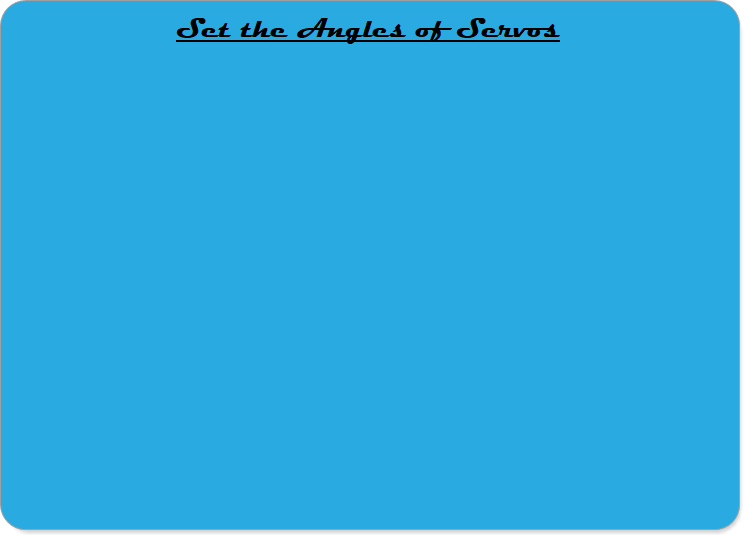Robotics 1
Kinematic Diagrams
A 'Kinematic Diagram' is a kind of drawing that is used in robotics to find the equations we need to control our robot manipulator.  In this video, you will build several different types of robotic manipulators, and learn how to draw kinematic diagrams matching the manipulators.  Then, you will learn how to draw frames on the kinematic diagrams following four rules known as the 'Denavit-Hartenberg' rules.Now that you know how to draw and build some different 2-DoF manipulators, you can use the PSoC 5 microcontroller to set the angles of the revolute joints.  In this video, you will learn how to use a 'PWM' block to set servo angles.To help you solidify your understanding and build your skills with kinematic diagrams, this video gives five examples of drawing Denavit-Hartenberg frames on 3-DoF manipulators.  The five examples cover the five 'standard manipulator types': (1) Cartesian, (2) Articulated, (3) SCARA, (4) Spherical, and (5) Cylindrical.
To complete this lab activity, make a video that includes the following in one video: